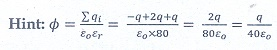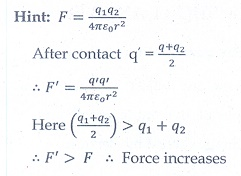Home | | Physics 12th Std | Electrostatics: Multiple choice questions with answers

# Electrostatics: Multiple choice questions with answers

Physics Book Back 1 mark questions with answers, solution - Physics : Electrostatics: Multiple choice questions with Answers, Solution and Explanation

Multiple choice questions

1. Two identical point charges of magnitude –q are fixed as shown in the figure below. A third charge +q is placed midway between the two charges at the point P. Suppose this charge +q is displaced a small distance from the point P in the directions indicated by the arrows, in which direction(s) will +q be stable with respect to the displacement?(a) A1 and A2

(b) B1 and B2

(c) both directions

(d) No stable

Hint: Since V=0 along equatorial line

2.Which charge configuration produces a uniform electric field?

(a) point Charge

(b) infinite uniform line charge

(c) uniformly charged infinite plane

(d) uniformly charged spherical shell

3.  What is the ratio of the charges |q1/q2for the following electric field line pattern?(a) 1/5

(b) 25/11

(c)  5

(d) 11/254.An electric dipole is placed at an alignment angle of 30º with an electric field of 2 × 105 N C-1. It experiences a torque equal to 8 N m. The charge on the dipole if the dipole length is 1 cm is

(a) 4 mC

(b) 8 mC

(c) 5 mC

(d) 7 mC5. Four Gaussian surfaces are given below with charges inside each Gaussian surface. Rank the electric flux through each Gaussian surface in increasing order.a.     D < C < B < A

b.     A < B = C < D

c.      C < A = B < D

d.     D > C > B > A6.The total electric flux for the following closed surface which is kept inside water(a)80q/ε

(b)q/40ε

(c)q/80ε

(d)q/160ε7. Two identical conducting balls having positive charges q1 and q2 are separated by a center to center distance r. If they are made to touch each other and then separated to the same distance, the force between them will be

a.     less than before

b.     same as before

c.      more than before

d.     zero8.Rank the electrostatic potential energies for the given system of charges in increasing order.(a) 1 = 4 < 2 < 3

(b) 2 = 4 < 3 < 1

(c) 2 = 3 < 1 < 4

(d) 3 < 1 < 2 < 49.  An electric field=10xexists in a certain region of space. Then the potential difference V = Vo – VA, where Vo is the potential at the origin and VA is the potential at x = 2 m is:

(a) 10 J

(b) – 20 J

(c) +20 J

(d) -10J10. A thin conducting spherical shell of radius R has a charge Q which is uniformly distributed on its surface. The correct plot for electrostatic potential due to this spherical shell isAns:b

11. Two points A and B are maintained at a potential of 7 V and -4 V respectively. The work done in moving 50 electrons from A to B is

a.     8.80 × 10-17 J

b.     -8.80 × 10-17 J

c.      4.40 × 10-17 J

d.     5.80 × 10-17 J12. If voltage applied on a capacitor is increased from V to 2V, choose the correct conclusion.

a.     Q remains the same, C is doubled

b.     Q is doubled, C doubled

c.      C remains same, Q doubled

d.     Both Q and C remain same13. A parallel plate capacitor stores a charge Q at a voltage V. Suppose the area of the parallel plate capacitor and the distance between the plates are each doubled then which is the quantity that will change?

a.     Capacitance

b.     Charge

c.      Voltage

d.    Energy density14. Three capacitors are connected in triangle as shown in the figure. The equivalent capacitance between the points A and C is(a) 1µF

(b) 2 µF

(c) 3 µF

(d)1/4µF15. Two metallic spheres of radii 1 cm and 3 cm are given charges of -1 x 10-2 C and 5 x 10-2 C respectively. If these are connected by a conducting wire, the final charge on the bigger sphere is

a.     × 10-2 C

b.     × 10-2 C

c.      × 10-2 C

d.     × 10-2 CTags : Physics , 12th Physics : Electrostatics
Study Material, Lecturing Notes, Assignment, Reference, Wiki description explanation, brief detail
12th Physics : Electrostatics : Electrostatics: Multiple choice questions with answers | Physics